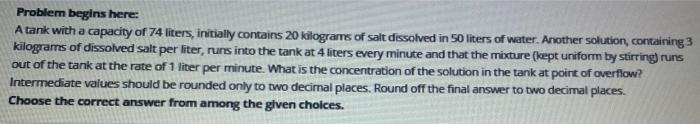# Question Solved1 AnswerProblem begins here: A tank with a capacity of 74 liters, initially contains 20 kilograms of salt dissolved in 50 liters of water. Another solution, containing 3 kilograms of dissolved salt per liter, runs into the tank at 4 liters every minute and that the mixture (kept uniform by stirring runs out of the tank at the rate of 1 liter per minute. What is the concentration of the solution in the tank at point of overflow? Intermediate values should be rounded only to two decimal places. Round off the final answer to two decimal places. Choose the correct answer from among the given choices.Transcribed Image Text: Problem begins here: A tank with a capacity of 74 liters, initially contains 20 kilograms of salt dissolved in 50 liters of water. Another solution, containing 3 kilograms of dissolved salt per liter, runs into the tank at 4 liters every minute and that the mixture (kept uniform by stirring runs out of the tank at the rate of 1 liter per minute. What is the concentration of the solution in the tank at point of overflow? Intermediate values should be rounded only to two decimal places. Round off the final answer to two decimal places. Choose the correct answer from among the given choices.
More
Transcribed Image Text: Problem begins here: A tank with a capacity of 74 liters, initially contains 20 kilograms of salt dissolved in 50 liters of water. Another solution, containing 3 kilograms of dissolved salt per liter, runs into the tank at 4 liters every minute and that the mixture (kept uniform by stirring runs out of the tank at the rate of 1 liter per minute. What is the concentration of the solution in the tank at point of overflow? Intermediate values should be rounded only to two decimal places. Round off the final answer to two decimal places. Choose the correct answer from among the given choices.